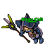## Wednesday, January 20, 2021

### TibiaGoals Riddles E5R4

Comment down below!

1.2.5+3=8 (You just move the arrow on number 2 from left to right)
Holistico

3.Make the 2 into a three by moving the vertical arrow in the bottom half from the left side to the right side!

Char: Hlys

4.By moving the bottom left arrow in the number 2 to the right side so it forms a number 3 instead.

-Essiee

5.5+3=8
Cambie la segunda flecha del número dos ponerla paralela y se vuelve 3
Aribebech

6.R= 5 + 3 = 8
Only move the arrow from west to east and now is 3 :p

Samuray de carro ;)

7.R= 5 + 3 = 8
Only move the arrow from west to east and now is 3 :p

Samuray de carro ;)

8.R= 5 + 3 = 8
Only move the arrow from west to east and now is 3 :p

Samuray de carro ;)

9.Change number 2 into number 3.
5+3=8

10.Change number 2 into number 3 by mowing vertical arrow from left to the right.
5+3=8
Char- Eternal Hunger
World- Antica

11.Change number 2 into number 3 by moving vertical arrow from left to the right.
5+3=8
Char- Eternal Hunger
World- Antica

12.Nehkz
Relania

Moving the very right arrow from the two (second from top) to the left side so it looks like a 3.

13.5+3 = 8
Sir wuachachucho

14.5+3=8
Vysterion

15.Character: Ragge Storm

You need to move one of the arrows of the "number 2", in particular, you need to move the "I" from the left to the right, then you will get 5 + 3 = 8.

16.5 + 3 = 8 (changing one arrow - making 3 from 2)

Shizurie

17.Oh no, I forgot the last one! Well, here's my answer to this one:
Move the lower left vertical arrow from the "2" to the right side of it to make it a "3".

18.you can move one arrow from the "2" (down left) to make it into "3" (down right)

Impure

19.1.Hello Akwariumelo, thank you for participating, you can still make riddles for fun, however you won't be considered in this event lottery, because you won't get 8/10 points anymore. Next event starts at 14.02.

20.21.5+3=8
Character name: Kutarate Fighter

22.Nehkz
Relania

Remove the very right arrow from the two and put it into the empty left spot so it looks like a 3. 5+3=8

23.Nehkz
Relania

Remove the very right arrow from the two and put it into the empty left spot so it looks like a 3. 5+3=8

24.Nehkz
Relania

Remove the very right arrow from the two and put it into the empty left spot so it looks like a 3. 5+3=8

25.5+3=8
Move one of the 2

26.Turn the 2 into 3
5 + 3 = 8

Char: Dyaniixz

27.5 + 3 = 8

Moving the arrow of the 2 to form a 3

Character: Marulina

28.5+3=8

PAMELIIN

29.30.Zuali

https://gyazo.com/04c0f669aec8c4a41d9ef5446c4bf0f5

31.changing the number 2 to 3 moving the lower vertical arrow (5+3 = 8)
Knight Narco

32.Character: Paollah

Just need to move the left bottom arrow of the number two to the right side modifying to 3!

33.Changing the arrow of the 2:

5+3=8

Dark Bombox <3

34.5+3=8 bebitha consentida

35.5+3=8
Nick: Renneus

1.Hello Renneus, thank you for participating, you can still make riddles for fun, however you won't be considered in this event lottery, because you won't get 8/10 points anymore. Next event starts at 14.02.

36.By moving one arrow on the 2 to make it 3.
5 + 3 = 8

Character: Elee Bae

37.You'll have to move the arrow that its on number 2 at the bottom left <, and move it to the right to make a number 3 >

Skyelie

38.39.5 + 3 = 8
moving the bottom left arrow of the "2" to the right of the same number, so the new equation would be " 5 + 3 = 8 " wich is true.

Character: Amy Meow

40.5 + 3 = 8
moving the bottom left arrow of the "2" to the right of the same number, so the new equation would be " 5 + 3 = 8 " wich is true.

Character: Amy Meow

41.Myo Disturbed

By moving the lower left arrow from the 2 and move it to the lower right spot to make it a 3 so it would be 5+3 = 8

42.5+3 = 8 make the 2 into a 3 EASSSSSY

43.Correct answer is indeed 5+3=8. Congratulations to all. We will update the list today and delete the people who wouldn't get 8/10 anymore. Those are: Alapontt, Bombillito, Charon line, Don Koperfildo, Goat Jogge, Kidler Zaklinacz, Letorwyn, Lysimachia, Madi Ati, Moonizera, Piixee, Prali Dreamcatcher, Sir Pitillox, Sir Wareono, Vapouryzer, Wedrujaca Nierzadnica, Zahiz and Zyakar. You can take part in this event, but you won't be considered in lottery anymore. You can try in next event which starts on 14.02. Thanks!

44.On the number 2, move the vertical line on the bottom (closest to the plus sign) over to the right to continue straight down from the other vertical line, thereby creating a number 3. Piixee

1.I'm sorry Piixee, but we accept the answers till server save. You still can take part in the event for fun, but sadly you didn't make it to make 8/10 correct answers to take part in the lottery. Thank you for participating though. Remember to join next event which starts on 14.02! <3